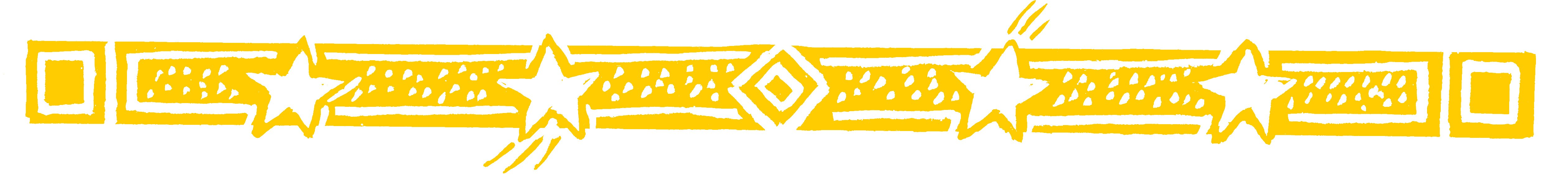ON A TRAIN

NOT-SO-LONG DIVISIONOn a long train journey?-  liven up your trip with this marvellous method for dividing numbers.

As in the case of ‘long’ multiplication ‘long’ division in this system is not long at all and, in fact, the answer to any division sum can be put down in one line.

• 369 ÷ 72 = 5 remainder 9

We use THE FIRST BY THE FIRST AND THE LAST BY THE LAST

Divide the 36 at the beginning of 369 by the first figure of 72: 36 ÷ 7 = 5 remainder 1.

This gives: 3619 ÷ 72 = 5

The remainder, 1, is placed as shown and makes 19 with the 9 following it.

From this 19 we subtract 2 × 5 (the answer figure multiplied by last figure of 72):
19 - 10 = 9, the remainder.

To sum up: for 369 ÷ 72:

36 ÷ 7 = 5 remainder 1 gives 3619 ÷ 72 = 5
and 19 - 2 × 5 = 9 the remainder,
so 3619 ÷ 72 = 5 rem 9

• Similarly 468 ÷ 73 = 6 remainder 30

Because 46 ÷ 7 = 6 remainder 4: 4648 ÷ 73 = 6,
then  48 - 3 ×  6 = 30, the remainder.

Question Comment !
 Tutorial Title Question Title No Questions 0 Questions Asked 0 Skipped Questions 0 Correctly Answered 0 Wrongly Answered 0 Total Question Attempts 0 Current Question Attempts 0

Skip Question

Reset Test

Halt Test

 No Questions 0 Questions Asked 0 Skipped Questions 0 Correctly Answered 0 Wrongly Answered 0 Total Question Attempts 0

Time Taken

CloseOn The Train

Similarly squares, squares roots are easily tackled (in one line) by the Vedic method. We can also solve equations and geometrical and trigonometrical problems. The Vedic system covers all areas of mathematics.

It is not possible to show all variations of the methods in this book: all the techniques shown can be extended in various ways.Number rules the universe
PYTHAGORAS